# Difference between revisions of "7"

## Summary

### Factorization

The number 7 is a prime number.

### Properties and families

Property or family Parameter values First few numbers Proof of satisfaction/membership/containment
prime number fourth prime number 2, 3, 5, 7, 11, 13, 17, 19, 23, 29, 31, 37, 41, 43, 47, 53, 59, 61, 67, 71, 73, 79, 83, 89, 97, [SHOW MORE]View list on OEIS divide and check
Mersenne number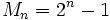, i.e.,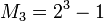plug and check
Mersenne prime (both a prime number and a Mersenne number) same as for Mersenne number combine above
safe prime (odd prime such that half of that minus one is also prime) second safe prime 5, 7, 11, 23, 47, 59, 83, 107, 167, [SHOW MORE]View list on OEIS plug and check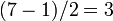is prime.
regular prime third regular prime 3, 5, 7, 11, 13, 17, 19, 23, 29, 31, 41, 43, 47, 53, 61, [SHOW MORE]View list on OEIS
twin prime (bigger member of pair) third twin prime, second bigger member twin prime Twin prime 7 - 2 = 5 is prime

## Structure of integers mod 7

### Discrete logarithm

3 is a primitive root mod 7, so we can take that as the base of the discrete logarithm. We thus get an explicit bijection from the additive group of integers mod 6 to the multiplicative group of nonzero congruence classes mod 7, given by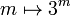. The inverse of that mapping is the discrete logarithm, i.e., the discrete logarithm ofismeans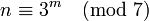:

Congruence class mod 7 (written as smallest positive integer) Congruence class mod 7 (written as smallest magnitude integer) Discrete logarithm to base 3, written as integer mod 6 Is it a primitive root mod 7 (if and only if the discrete log is relatively prime to 6)? Is it s quadratic residue or nonresidue mod 7 (residue if discrete log is even, nonresidue if odd)
1 1 0 No quadratic residue
2 2 2 No quadratic residue
3 3 1 Yes quadratic nonresidue
4 -3 4 No quadratic residue
5 -2 5 Yes quadratic nonresidue
6 -1 3 No quadratic nonresidue
-- -- -- Total: 2 Yes, 4 No,is Euler totient function
Total: 3 residues, 3 nonresidues. Equal number of both

Alternatively, we could take discrete logs to base 5, which is the other primitive root. This is simply the negative of the other discrete log:

Congruence class mod 7 (written as smallest positive integer) Congruence class mod 7 (written as smallest magnitude integer) Discrete logarithm to base 5, written as integer mod 6 Is it a primitive root mod 7 (if and only if the discrete log is relatively prime to 6)? Is it s quadratic residue or nonresidue mod 7 (residue if discrete log is even, nonresidue if odd)
1 1 0 No quadratic residue
2 2 4 No quadratic residue
3 3 5 Yes quadratic nonresidue
4 -3 2 No quadratic residue
5 -2 1 Yes quadratic nonresidue
6 -1 3 No quadratic nonresidue
Total: 2 Yes, 4 No,is Euler totient function
Total: 3 residues, 3 nonresidues. Equal number of both

## Primitive root theory

### Primitive roots

The number of primitive roots equals the number of generators of the additive group of integers modulo 6 (= 7 - 1) which is the Euler totient function of 6, which is 2. Ifis a primitive root, the primitive roots areand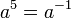.

Explicitly, the primitive roots are 3 and 5 (= -2).

### Significance of 10 being a primitive root

If 10 is a primitive root modulo a prime, then the primeis a full reptend prime in base 10, i.e., the decimal expansion ofhas a repeating block of the maximum possible length. The condition holds for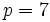(note that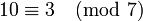and 3 is a primitive root), and the corresponding decimal expansion of 1/7 is:The corresponding number: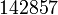has the property that it is a cyclic number, i.e., its product with any of the numbers from 1 to 6 is obtained by cyclically permuting its digits.

Of the six congruence classes of invertible elements mod 7, three are quadratic residues and three are quadratic nonresidues. Ifis a primitive root, the quadratic nonresidues are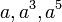, and the quadratic residues are. Alternatively, we can obtain the quadratic residues by taking the congruence classes of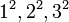.

Explicitly, the quadratic residues are 1,2,4 and the quadratic nonresidues are 3,5,6.

If we also choose to include 0, the quadratic residues are 0,1,2,4 and the quadratic nonresidues are 3,5,6.

Fill this in later

### Condition for 7 to be a quadratic residue modulo a prime

Whether or not 7 is a quadratic residue modulo a given prime depends only on the congruence class of that prime modulo 28. This follows from quadratic reciprocity (applicable only to odd primes, but the case of 2 is isolated), which states that: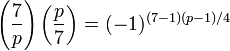We note that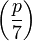depends only on the congruence class ofmod 7, and the right side depends only on the congruence class ofmod 4. Thus,depends only on the congruence class ofmod 28.

The full list is below:

Fill this in later

By Dirichlet's theorem on arithmetic progressions, we thus find that there are infinitely many primesfor which 7 is a quadratic residue and infinitely many primesfor which 7 is a quadratic nonresidue.

## Cyclotomic theory

### Cyclotomic extension of primitive roots of unity

Fill this in later

### Constructibility of regular 7-gon

7 is the smallest primefor which the regular-gon is not constructible by straightedge and compass. In fact, it is the smallest natural numberfor which the regular-gon is not constructible by straightedge and compass. This can be seen by noting that the cyclotomic extension of adjoining 7th roots is a degree six Galois extension and cannot be expressed using successive quadratic extensions.